# A Gentle Introduction to Vector Databases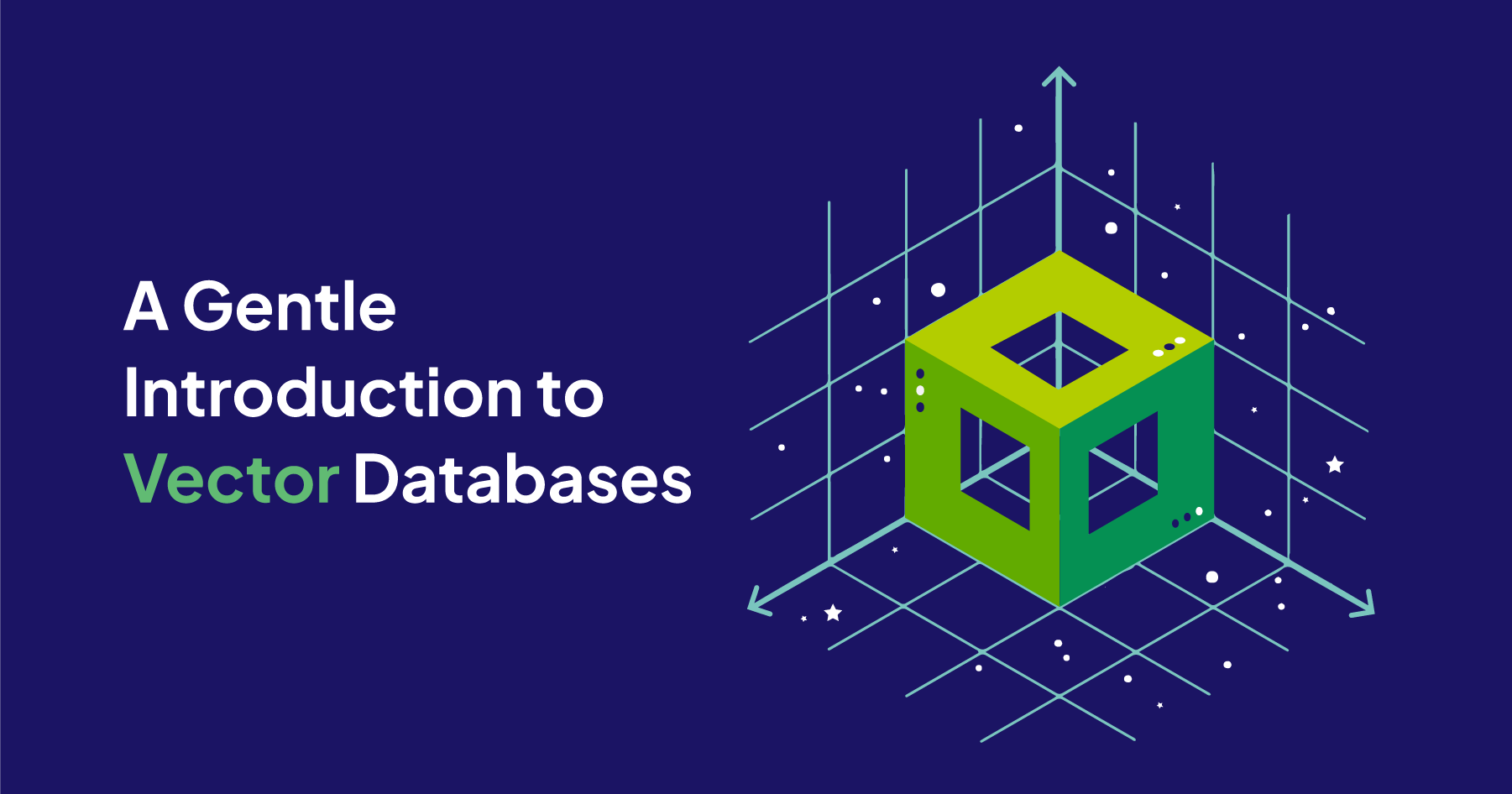If you have just recently heard the term “vector database” for the first time, you are not alone. Although vector databases have been around for a few years, they’ve just recently drawn the wider developer community’s attention.

The excitement around vector databases is closely related to the release of ChatGPT. Since late 2022, the public has started to understand the capabilities of state-of-the-art large language models (LLMs), while developers have realized that vector databases can enhance these models further.

This article will walk through what vector databases are and explain some of their core concepts, such as vector embeddings and vector search. Then we will dive into the technical details of how distance metrics are used in vector search and how vector indexes enable efficient retrieval. Once we have a fundamental understanding of vector databases, we will discuss their use cases and the current tool landscape.

## What is a Vector Database?​

A vector database indexes, stores, and provides access to structured or unstructured data (e.g., text or images) alongside its vector embeddings, which are the data's numerical representation. It allows users to find and retrieve similar objects quickly at scale in production.This section introduces the core concepts of vector databases. We will discuss what vector embeddings are, how they enable similarity search, and how they accelerate it.

### Vector Embeddings​

When you think of data, you probably think of neatly organized numbers in spreadsheets. This type of data is called structured data because it can easily be stored in tabular format. But roughly 80% of today’s data is said to be unstructured. Examples of unstructured data are images, text (e.g., documents, social media posts, or emails), or time series data (e.g., audio files, sensor data, or video). The problem with unstructured data is that it is difficult to store it in an organized way so you can easily find what you are looking for.

But innovations in Artificial Intelligence (AI) and Machine Learning (ML) have enabled us to numerically represent unstructured data without losing its semantic meaning in so-called vector embeddings. A vector embedding is just a long list of numbers, each describing a feature of the data object.

An example is how we numerically represent colors in the RGB system, where each number in the vector describes how red, green, or blue a color is. E.g., the following green color can be represented as [6, 205, 0] in the RGB system.But fitting more complex data, such as words, sentences, or text, into a meaningful series of numbers isn’t trivial. This is where ML models come in: ML models enable us to represent the meaning of, e.g., a word as a vector because they have learned to represent the relationship between different words in a vector space. These types of ML models are also called embeddings model or vectorizer.

Below you can see an example of the three-dimensional vector space of the RGB system with a few sample data points (colors).Depending on the ML model used, the data can be represented in different vector spaces. Vector spaces can differ in the number of dimensions ranging from tens to thousands, where the precision of the representation increases with increasing dimensions. In the color example, there are also different ways to represent a color numerically. E.g., you can represent the previous green color in the RGB system as [6, 205, 0] or in the CMYK system as [97, 0, 100, 20].

This is why it is important to use the same vectorizer for all your data to ensure it is in the respective vector space.

Vector embeddings numerically capture the semantic meaning of the objects in relation to other objects. Thus, similar objects are grouped together in the vector space, which means the closer two objects, the more similar they are.

For now, let’s consider a simpler example with numerical representations of words - also called word vectors. In the following image, you can see the words “Wolf” and “Dog” close to each other because dogs are direct descendants of wolfs. Close to the dog, you can see the word “Cat,” which is similar to the word “Dog” because both are animals that are also common pets. But further away, on the right-hand side of the illustrated vector space, you can see words that represent fruit, such as “Apple” or “Banana”, which are close to each other but further away from the animal terms.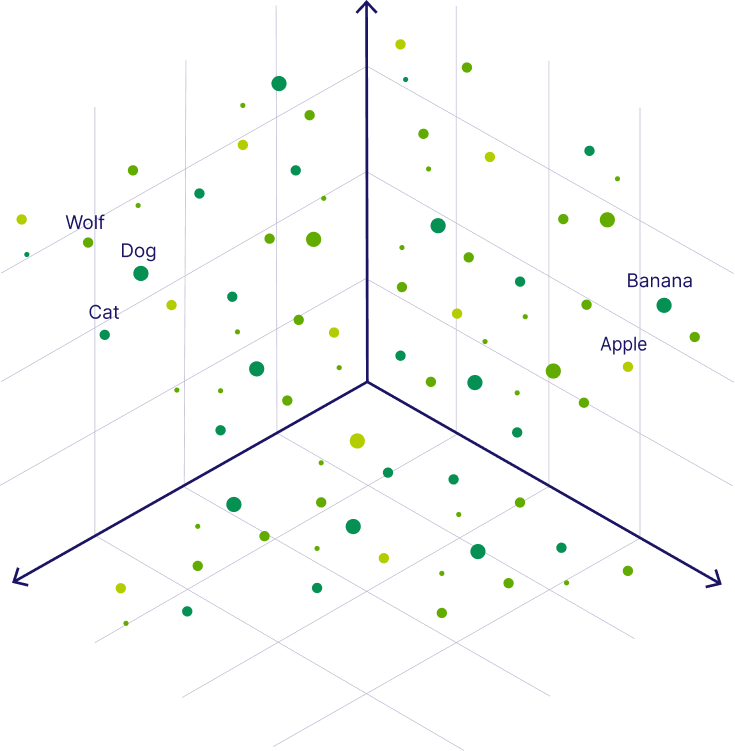For an in-depth explanation of vector embeddings, see Vector Embeddings Explained

Vector embeddings allow us to find and retrieve similar objects from the vector database by searching for objects that are close to each other in the vector space, which is called vector search, similarity search, or semantic search.

Similarly to how we can find similar vectors for a dog, we can find similar vectors to a search query. E.g. to find words similar to the word “Kitten”, we can generate a vector embedding for the word “Kitten” and retrieve all items close to the query vector, such as the word “Cat”, as illustrated below.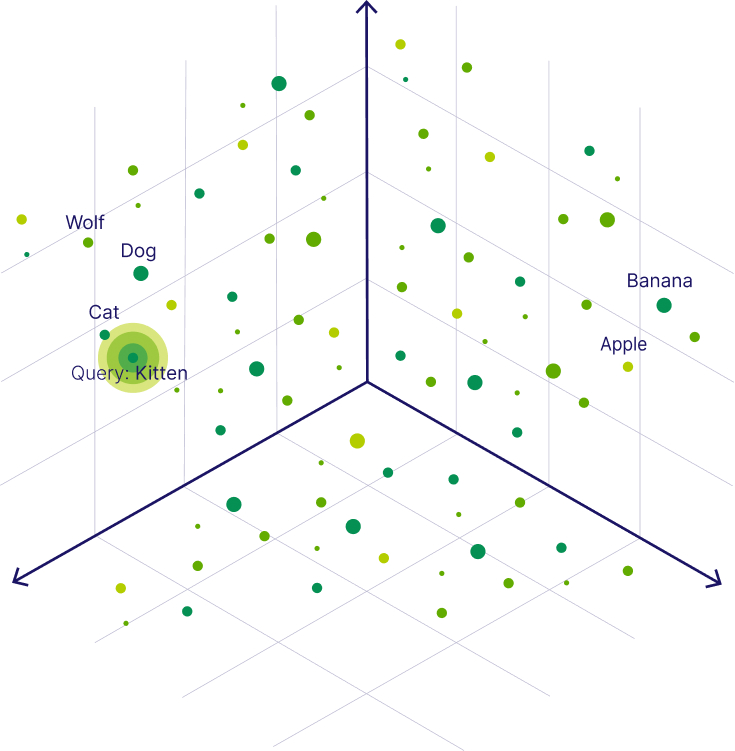The numerical representation of a data object allows us to apply mathematical operations to them, such as calculating the distance between two vector embeddings to determine their similarity. To calculate the distance between two vectors, you can use several similarity measures. E.g., Weaviate supports the following distance metrics:

• Squared Euclidean or L2-squared distance calculates the straight-line distance between two vectors. Its range is [0, ∞], where 0 represents identical vectors, and larger values represent increasingly dissimilar vectors.
• Manhattan or L1 distance calculates the sum of the lengths of the projections of the line segment between the points onto the coordinate axes. Its range is [0, ∞], where 0 represents identical vectors, and larger values represent increasingly dissimilar vectors.
• Cosine similarity calculates the cosine of the angle between two vectors. In Weaviate, the cosine distance is used for the complement of cosine similarity. Its range is [0, 2], where 0 represents identical vectors, and 2 represents vectors that point in opposite directions.
• Dot product calculates the product of the magnitudes of two vectors and the cosine of the angle between them. Its range is [-∞, ∞], where 0 represents orthogonal vectors, and larger values represent increasingly similar vectors. In Weaviate, the negative dot product is used to keep the intuition that larger values represent increasingly dissimilar vectors.
• Hamming distance calculates the number of differences between vectors at each dimension.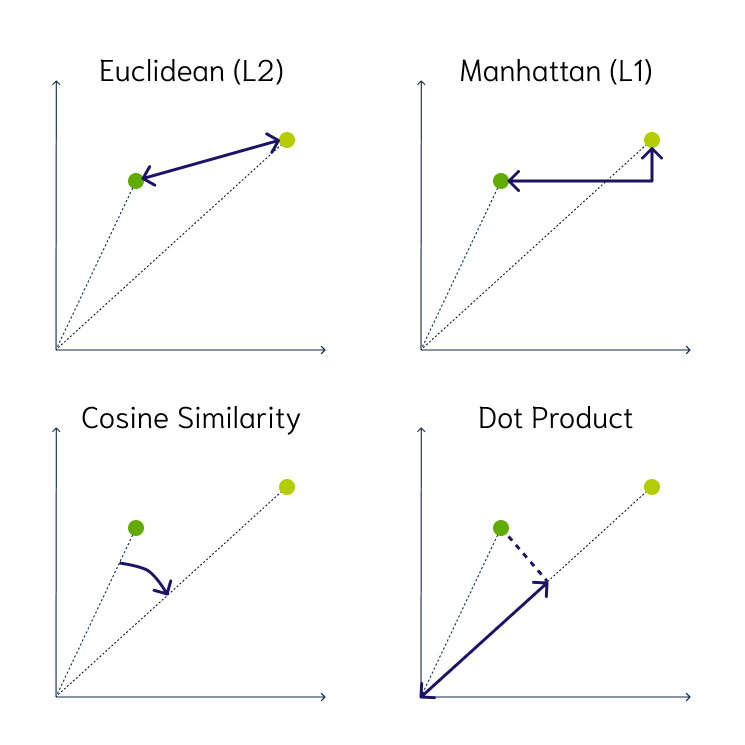As you can see, there are many different similarity measures. As a rule of thumb, select the same metric as the one used to train the ML model. If you are unsure, which one was used, you can usually find this information on the hosting service’s site, e.g., OpenAI’s ada-002 uses cosine similarity or MiniLM hosted on Hugging Face supports cosine similarity, dot product, and Euclidean distance.

To learn more about the different distance metrics, you can continue reading our blog post on What are Distance Metrics in Vector Search?

### Vector Indexing for Approximate Nearest Neighbor Approach​

Vector indexing is the process of organizing vector embeddings in a way that data can be retrieved efficiently.

When you want to find the closest items to your query vector, the brute force approach would be to use the k-Nearest Neighbors (kNN) algorithm. But calculating the similarity between your query vector and every entry in the vector database can become computationally expensive if you have millions or even billions of data points because the required calculations increase linearly (O(n)) with the dimensionality and the number of data points.

A more efficient solution to find similar objects is to use an approximate nearest neighbor (ANN) approach. The underlying idea is to pre-calculate the distances between the vector embeddings and organize and store similar vectors close to each other (e.g., in clusters or a graph), so that you can later find similar objects faster. This process is called vector indexing. Note, that the speed gains are traded in for some accuracy because the ANN approach returns only the approximate results.

In the previous example, one option would be to pre-calculate some clusters, e.g., animals, fruits, and so on. So, when you query the vector database for "Kitten", you could start your search by only looking at the closest animals and not waste time calculating the distances between all fruits and other non-animal objects. More specifically, the ANN algorithm can help you to start the search in a region near, e.g., four-legged animals. Then the algorithm would also prevent you from venturing further from relevant results, as the objects are pre-organized by similarity.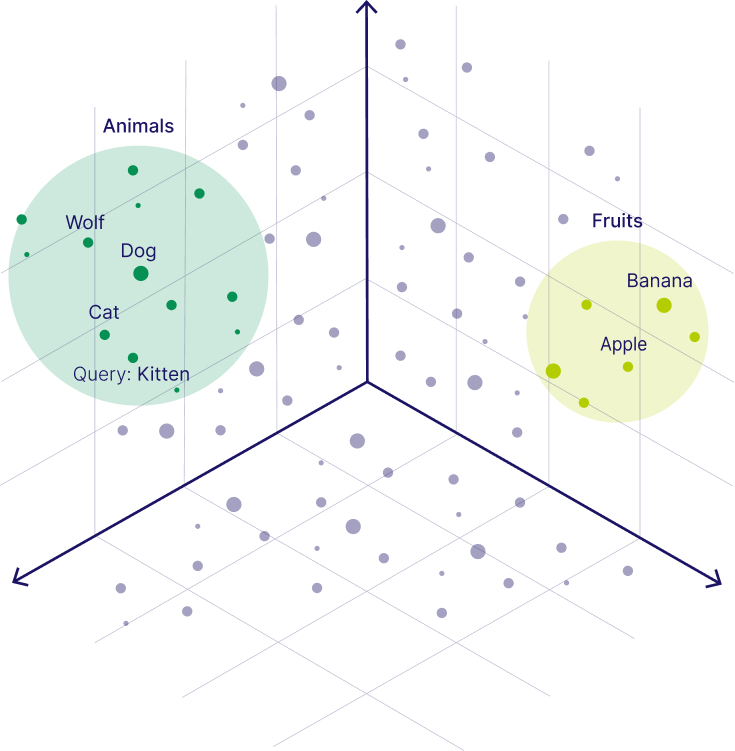The above example roughly describes the concept of the Hierarchical Navigable Small World (HNSW) algorithm, which is the default ANN algorithm in Weaviate. However, there are several ANN algorithms to index the vectors, which can be categorized into the following groups:

• Clustering-based index (e.g., FAISS)
• Proximity graph-based index (e.g., HNSW)
• Tree-based index (e.g., ANNOY)
• Hash-based index (e.g., LSH)
• Compression-based index (e.g., PQ or SCANN)

Note that indexing enables fast retrieval at query time, but it can take a lot of time to build the index initially.

Further reading Why Is Vector Search So Fast?

## Use Cases of Vector Databases​

Vector databases’ search capabilities can be used in various applications ranging from classical ML use cases, such as recommender systems, to providing long-term memory to large language models in modern applications.

The most popular use case of vector databases is for search. Because a vector database can help find similar objects, it is predestined for applications where you might want to find similar products, movies, books, songs, etc. That’s why vector databases are also used in recommendation systems in classical ML applications as a restated task of search.

With the rise of LLMs, vector databases have already been used to enhance modern LLM applications. You might have already come across the term that vector databases provide LLMs with long-term memory. LLMs are stateless, which means that they immediately forget what you have just discussed if you don’t store this information in, e.g., a vector database and thus provide them with a state. This enables LLMs to hold an actual conversation. Also, you can store the domain-specific context in a vector database to minimize the LLM from hallucinating. Other popular examples of vector databases improving the capabilities of LLMs are question-answering and retrieval-augmented generation.

Here is an example demo called HealthSearch of a Weaviate vector database in action together with an LLM showcasing the potential of leveraging user-written reviews and queries to retrieve supplement products based on specific health effects.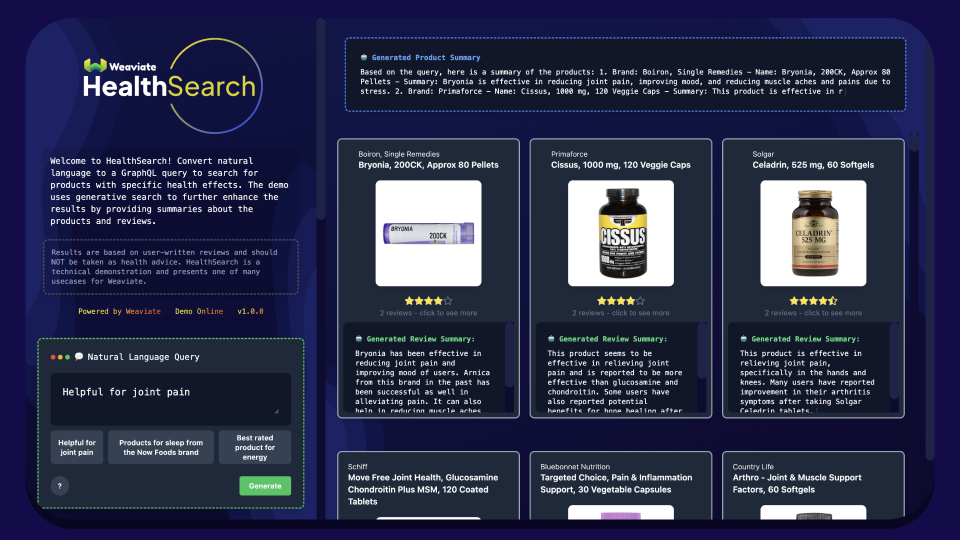## Tool Landscape around Vector Databases​

Although vector databases are AI-native and specifically designed to handle vector embeddings and enable efficient vector search, alternatives like vector libraries and vector-capable databases exist as well.

### Vector Database vs. Traditional (Relational) Database​

The main difference between a traditional (relational) database and a modern vector database comes from the type of data they were optimized for. While a relational database is designed to store structured data in columns, a vector database is also optimized to store unstructured data (e.g., text, images, or audio) and their vector embeddings.

Because vector databases and relational databases are optimized for different types of data, they also differ in the way data is stored and retrieved. In a relational database, data is stored in columns and retrieved by keyword matches in traditional search. In contrast, vector databases also store the vector embeddings of the original data, which enables efficient semantic search.

For example, imagine you have a database that stores Jeopardy questions, and you want to retrieve all questions that contain an animal. Because traditional search relies on keyword matches, you would have to create a big query that queries all animals (e.g., contains "dog", or contains "cat", or contains "wolf", etc.). With semantic search, you could simply query for the concept of "animals".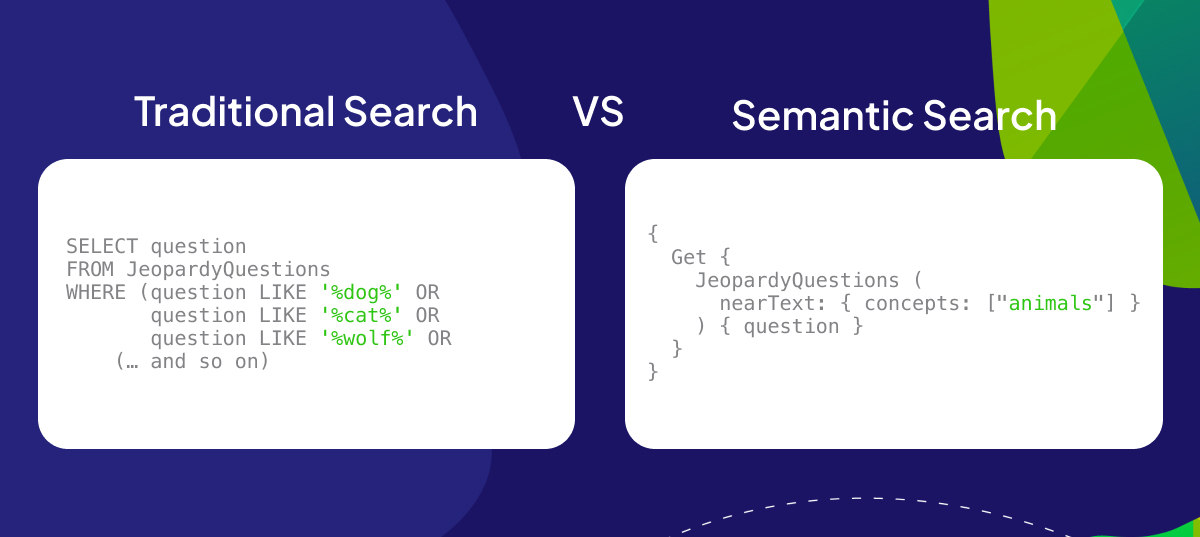Because most vector databases do not only store vector embeddings but store them together with the original source data, they are not only capable of vector search but also enable traditional keyword search. Some vector databases like Weaviate even support hybrid search capabilities which combine vector search with keyword search.

### Vector Database vs. Vector-Capable Database (SQL and NoSQL)​

Today many existing databases have already enabled vector support and vector search. However, they usually don’t index the vector embeddings, which makes the vector search slow. Thus, an advantage of AI-native vector databases over vector-capable databases is their efficiency in vector search due to vector indexing.

### Vector Database vs. Vector Indexing Library​

Similarly to vector databases, vector libraries also enable fast vector search. However, vector libraries only store vector embeddings of data objects, and they store them in in-memory indexes. This results in two key differences:

1. Updatability: The index data is immutable, and thus no real-time updates are possible.
2. Scalability: Most vector libraries cannot be queried while importing your data, which can be a scalability concern for applications that require importing millions or even billions of objects.

Thus, vector libraries are a great solution for applications with a limited static snapshot of data. However, if your application requires real-time scalable semantic search at the production level, you should consider using a vector database.

## Summary​

This article explained that vector databases are a type of database that indexes, stores, and provides access to structured or unstructured data alongside its vector embeddings. Vector databases like Weaviate allow for efficient similarity search and retrieval of data based on their vector distance or similarity at scale.

We covered core concepts around vector databases, such as unstructured data and vector embeddings, and discussed that vector databases enable efficient vector search by leveraging ANN algorithms. Additionally, we explored the tool landscape around vector databases and discussed the advantages of vector databases over traditional and vector-capable databases and vector libraries.

## What's next​

Check out Getting Started with Weaviate, and begin building amazing apps with Weaviate.

You can reach out to us on Slack or Twitter, or join the community forum.

Weaviate is open source, and you can follow the project on GitHub. Don’t forget to give us a ⭐️ while you are there!